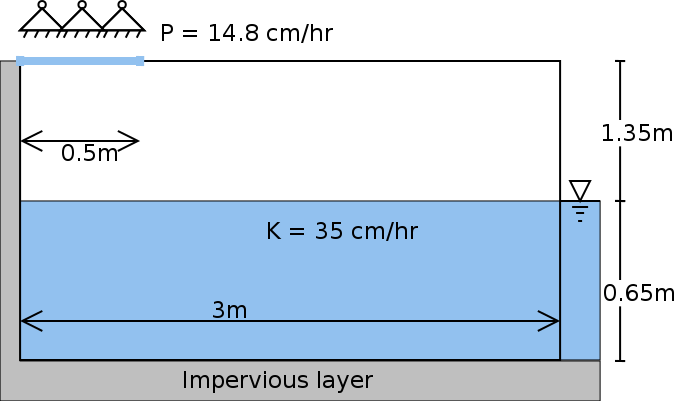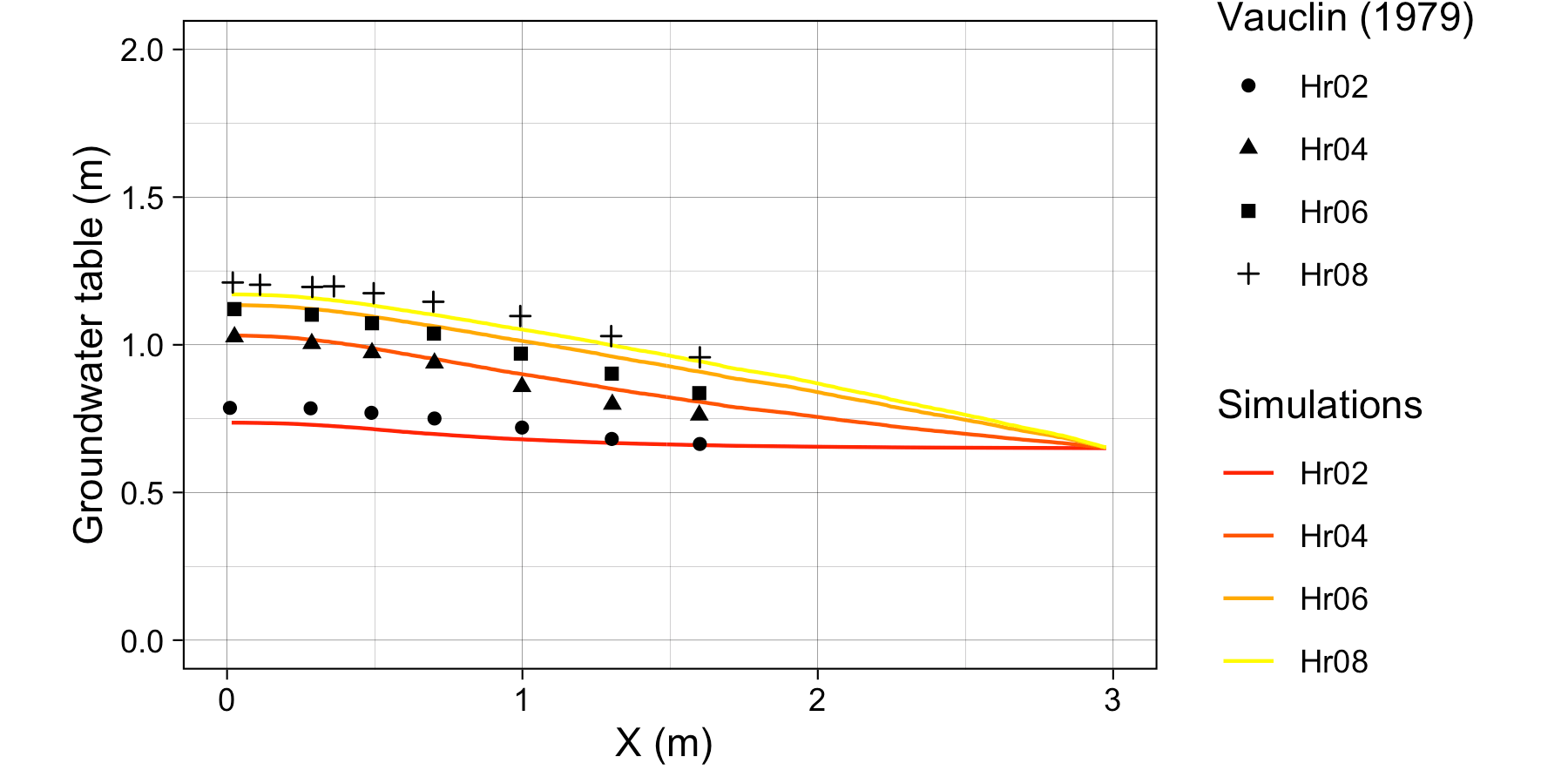# Vauclin沙箱试验模拟Vauclin’s experiment [@Vauclin1979] is designed to assess groundwater table change and soil moisture in the unsaturated layer under precipitation or irrigation. The experiment was conducted in a sandbox with dimension 3 m long $\times$ 2 m deep $\times$ 0.05 m wide. The box was filled with uniform sand particles with measured hydraulic parameters: the saturated hydraulic conductivity was $35 cm/hr$ and porosity was $0.33$ m$^3$/m$^3$. The left and bottom of the sandbox were impervious layers, and the top and the right side were open. A hydraulic head was set constant at $0.65 m$. Constant irrigation ($1.48$ cm/hr) was applied over the first $50$ cm of the top-left of the sandbox while the rest of the top was covered to avoid water loss via evaporation.The experiment’s initial condition is an equilibrium water table under constant hydraulic head from the right side. That is, the saturated water table across the sandbox was kept stable at $0.65$ m. When the groundwater table reached equilibrium, irrigation was initiated at $t = 0$. The groundwater table was then measured at 2, 4, 6, and 8 hours at several locations along the length of the box.

Besides the parameters specified in [@Vauclin1979], additional information is needed by the SHUD, including the $\alpha$ and $\beta$ in the van Genutchen equation and water content ($\theta _s$). Therefore, we use a calibration tool to estimate the representative values of these parameters. The use of calibration in this simulation is reasonable because the model – inevitably – simplifies the real hydraulic processes. The calibration thus nudges the parameters to representative values that approach or fit the true natural processes. The calibrated values are $\theta _s = 0.32 m^3/m^3$, $\alpha = 6.0$ and $\beta = 6.0$. Like the simulated results in [@Vauclin1979] and [@Shen2010], a mismatch exists between the simulations and measurements. This mismatch may be due to (1) the aquifer description of unsaturated and saturated layers limiting the capability to simulate infiltration and recharge in the unsaturated zone, or (2) the horizontal unsaturated flow assumptions no longer hold at the relatively microscopic scales of this experiment.

The SHUD simulated the groundwater table at all four measurement points (see Figure). The maximum bias between simulation and Vauclin’s observations is $4.6cm$, with $R^2$ = $0.99$. When the calibration takes more soil parameters into account, the bias in simulation decreases to $3 cm$. Certainly, the simplifications employed by SHUD for the unsaturated and saturated zone benefits the computation efficiency while limiting the applicability of the model for micro-scale problems.

The simulations, compared against Vauclin’s experiment, validate the algorithm for infiltration, recharge, and lateral groundwater flow. More reliable vertical flow within unsaturated layer requires multiple layers, which is planned in next version of SHUD.